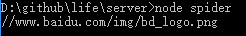# NodeJs实现爬虫功能

2017年01月20日Web后端0

## 实现爬取数据功能

``````var http = require('http');

var req = http.get('http://www.baidu.com', function(res) {
var html = '';
res.on('data', function(chunk) {
html += chunk;
});
res.on('end', function() {
console.log(html);
});
});``````

## 解析数据

``npm install cheerio --save``

``````res.on('end', function() {
\$ = cheerio.load(html);   // 将HTML DOM传给cheerio
console.log(\$('#lg > img').attr('src'));
});``````## 下载资源

``````var http = require('http');
var fs = require("fs");
var cheerio = require('cheerio');

var req = http.get('http://www.baidu.com', function(res) {
var html = '';
res.on('data', function(chunk) {
html += chunk;
});
res.on('end', function() {
var oImg = \$('img'),
length = oImg.length;
for (var i = 0; i < length; ++i) {
getPic(oImg.eq(i).attr('src'), 'pic/' + i + '.png');
console.log(oImg.eq(i).attr('src'));  // 打印img路径，注1.
}
});
});

// 下载图片函数
function getPic(url, local) {
http.get(url, function(res) {
var img = '';
res.setEncoding("binary"); // 必须设置response的编码为binary，否则下载下来的图片会打不开
res.on('data', function(chunk) {
img += chunk;
});
res.on('end', function() {
fs.writeFile(local, img, 'binary', function(err) { // 写操作函数
if (err) {
} else {
console.log('sucess!');
}
});
});
});
}``````